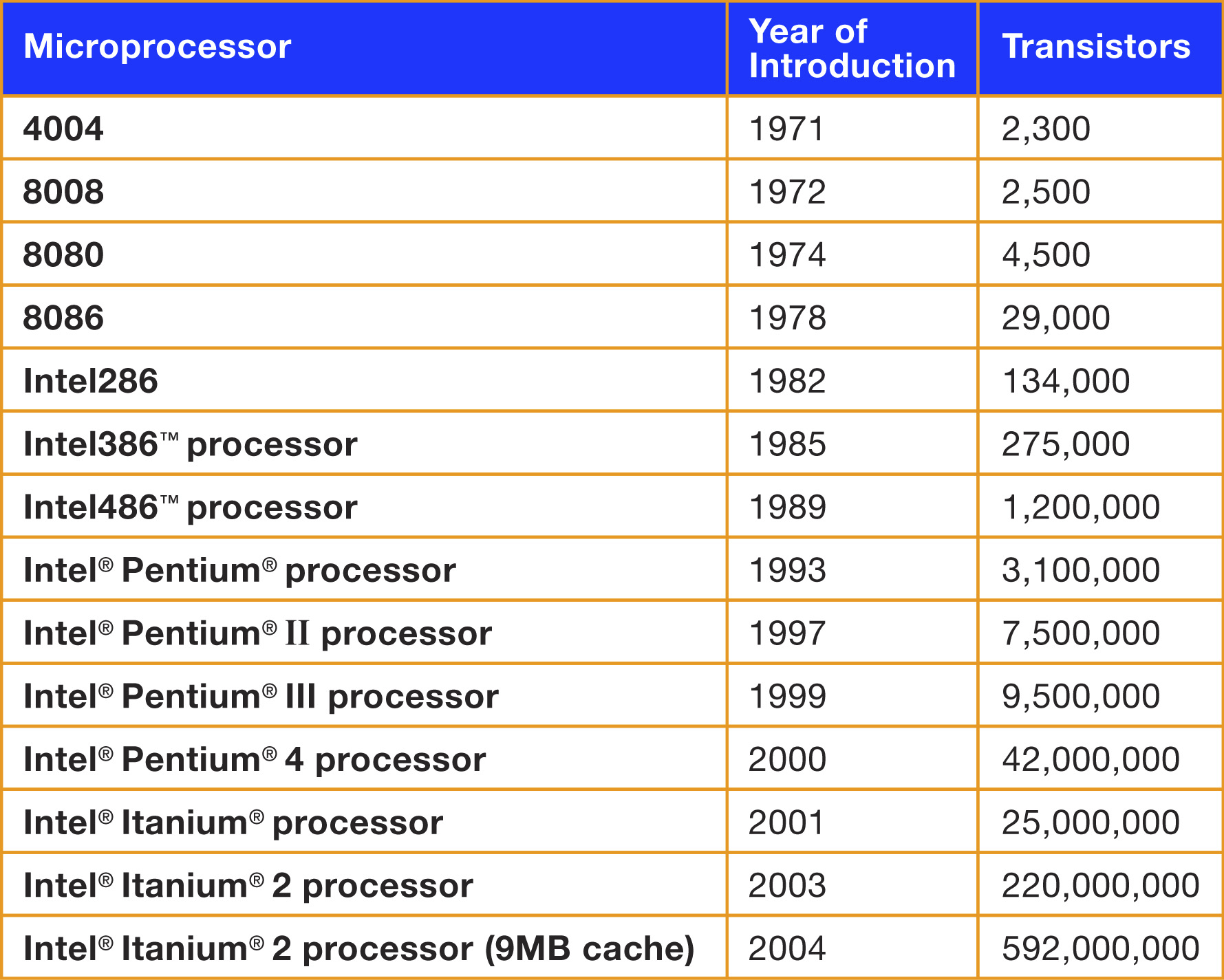December 10, 2019

## How cells grow

Cells in a culture grow every day

We want to know the number of cells every day: ncell[t]

Here t is the time in days.

We start with an initial number of cells, that we call initial

Each day, the number of cells increases by a factor rate

$\texttt{ncell[t]} = \texttt{initial} \cdot \texttt{rate}^\texttt{t}$

## Graphically

plot(t, ncell)We cannot see what happens when values are small

## Logarithmic scale (“semi-log”)

plot(ncell ~ t, log="y")We can see better using a logarithmic vertical scale

## Size of the culture

When the cells grow in a petri dish, then the culture will form a circle

The area of the circle is proportional to the number of cells.

Thus, the circle radius changes with time following this equation $\texttt{ncell[t]}=K \texttt{r}^2$ in other words $\texttt{r}=\sqrt{\frac{\texttt{ncell[t]}}{K}}$

## Log-log scale

par(mfrow=c(1,2))
plot(radius ~ ncell, log="xy")## Kleiber’s Law

### (Physiological Reviews 1947 27:4, 511-541)

Relation of Body size v/s metabolic rate## Same in log-log scale## We saw the same in Homework 5

plot(income~population, world)## Using log-log scale

plot(income~population, world, log="xy")## Same, with colors and point size

plot(income~population, world, log="xy", pch=16,
col=region, cex = 3*sqrt(area/max(area))+0.1)## Moore’s Law

A idea from ~1970, by George Moore (Intel)

The simple version of this law states that processor speeds will double every two years

More specifically, “the number of transistors on a CPU would double every two years”

(see paper)## Intel says## Real data: Number of transistors in chips v/s year

plot(count~Date, data=trans)## Semilog scale: Number of transistors v/s year

plot(count ~ Date, data=trans, log="y")## Same happens with DNA

### Cost of sequencing human genome## Cost of sequencing human genome

### Months since Sept 2000## The Robots Are Coming

### John Lanchester

• 1992 Russo-American moratorium on nuclear testing
• 1996 Computer simulations to design new weapons
• Needed more computing power than could be delivered by any existing machine

## The Robots Are Coming

### John Lanchester

• USA designed ASCI Red, the first supercomputer doing over one teraflop
• ‘flop’ is a floating point operation (multiplication) per second
• teraflop is $$10^{12}$$ flops
• In 1997, ASCI Red did 1.8 teraflops
• The most powerful supercomputer in the world until about the end of 2000.

## The Robots Are Coming

In his book, John Lanchester says

"I was playing on Red only yesterday – I wasn’t really, but I did have a go on a machine that can process 1.8 teraflops.

"This Red equivalent is called the PS3: it was launched by Sony in 2005 and went on sale in 2006.

## The Robots Are Coming

"Red was [the size of] a tennis court, used as much electricity as 800 houses, and cost US\$55 million. The PS3 fits under the TV, runs off a normal power socket, and you can buy one for £200.

"[In 10 years], a computer able to process 1.8 teraflops went from being something that could only be made by the world’s richest government […], to something a teenager could expect [as a gift].